# AP Computer Science A : Standard Data Structures

## Example Questions

← Previous 1 3 4 5 6 7 8 9 10 11

### Example Question #1 : Int

int x = 10;

int y = 4;



int z = x / y;

What is the value of z?Explanation:

There are two reasons why this will not produce the expected output, 2.5:

1. When dividing by two integer operands, the result will be returned as an int. Solve this by casting either of the operands (or the entire expression) to the float or double type.
2. When storing a floating-point value in an integer variable, the decimal is truncated (discarded, cut off, not considered). Even if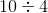was configured as suggested in step 1, and returned 2.5, the value 2 would be the value stored in z. Solve this value by declaring z as a float.

### Example Question #1 : Standard Data Structures

Consider the following code:

int i = 55, j = 3;

System.out.println((i / j + 3) / 5);

What is the output for the code above?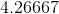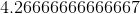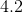There is an error in the code.Explanation:

Be careful! This is integer division.  Therefore, for every division, you will lose your decimal place.  Thus:

i / j is the same as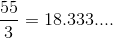, but it becomes.

Then, you will have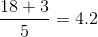.  Once more, you lose the decimal and getas your result.

### Example Question #1 : Standard Data Structures

Which of the following gurantees that your division does not lose its decimal portion?

None of the answers are correct.

double d = (double)(55/3);

double d = (double)((int)55 / (int) 3);

double d = 55 / 3;

double d = 1.0 * 55 / 3;

double d = 1.0 * 55 / 3;

Explanation:

Remember that so long as one element of a set of multiplications / divisions is a floating point value, the whole thing will be a floating point value.  Thus, the correct answer is:

double d = 1.0 * 55 / 3;

You have to evaluate the right side:

1.0 * 55 will become 55.0.  This will then finish out as a double in the division and store that value in d.

Note that the following does not work:

double d = (double)(55 / 3);

This code will first do the integer division 55 / 3.  This will resolve to 18.  Only then will the double cast occur.  This will give you 18.0 but not 18.33333...

### Example Question #1 : Int

Consider the following code:

int i = 100;

double d = 4.55, d2 = 3.75;

int j = (int)(d * 100 + d2);

What is the value of j at the end of the code's execution?

411

459

403

399

458

458

Explanation:

Do not over think this.  Begin by evaluating the expression:

d * 100 + d2

This is the same thing as: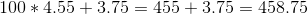Now, this value is then cast to an integer:

(int)(458.75)

Remember that when you type cast an integer from a double value, you drop the decimal place completely.  You do not round up or down.  You just truncate it off.  Thus, the answer is 458.

### Example Question #51 : Computer Science

Consider the following code:

int i = 3;

for(int j = 5; j > 0; j--) {

i += i;

}

What will be the value of i at the end of this loop's iteration?

729

96

48

243

24

96

Explanation:

The loop in question executes for j values of 5 through 1.  Thus, you will execute 5 times.  For each looping, then you will have:

i = 3 + 3 = 6

i = 6 + 6 = 12

i = 12 + 12 = 24

i = 24 + 24 = 48

i = 48 + 48 = 96

### Example Question #1 : Primitive Data Types

Consider the code below:

int i = 5, p = 27;

for(int l = 23; l < p; l++) {

     i *= (l - 22);

}

What is the value for i at the end of the code above?

5

120

150

75

0

120

Explanation:

You could always trace the loop in the code manually. You know that it is going to run from l = 23 to l = 26.  Recall that *= could be rewritten:

i = i * (l - 22)

Now, let's consider our first looping. For this, we would have:

i = 5 * (23 - 22) = 5 * 1

Now, let's calculate i for each looping from 23 to 26:

23: 5

24: 5 * (24 - 22) = 5 * 2 = 10

25: 10 * (25 - 22) = 10 * 3 = 30

26: 30 * (26 - 22) = 30 * 4 = 120

### Example Question #1 : Primitive Data Types

Consider the code below:

int val = 205;

for(int i = 0; i < 5; i++) {

val /= 2;

}

At the end of its execution, what is the value of the variable val in the code above?

6.40625

25

5.90225

12

6

6

Explanation:

Recall that the operator /= could be rewritten:

val = val / 2;

Now, recall also that integer division drops the decimal portion always. Therefore, this program will loop 5 times, doing the division of val by 2 each time. This gives you: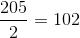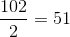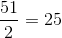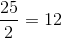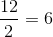### Example Question #1 : Primitive Data Types

Which of the following variable assignments is NOT a valid assignment statement?

a. 15 = x - 15;

b. num = x * y;

c. x = y + 15

d. x + y = 15

b, c

d

d, c

a, d

a, d

Explanation:

a. 15 = x - 15;

You can not assign a value to an integer because they are not variables and do not store information.

d. x + y = 15

This statement does not assign a value to a single integer, therefore, it is not a valid variable assignment.

### Example Question #2 : Standard Data Structures

How do we set a method to return an Int in Swift(iOS)?

func method->Int{}

method->Int {}

func() -> Int{}

func method() -> Int {}

func method() -> Int {}

Explanation:

In Swift, all methods must first say what they are. They are functions, so they are prefixed with func. Next, methods must have a name. In this case, we named it method. All methods need to specify parameters, even if there are no parameters. So, method() i.e. no parameters. Finally, we wanted to return an Int. So we set the return type using -> Int.

### Example Question #2 : Standard Data Structures

How do we set a method to return an Int in Swift(iOS)?

func method->Int{}

method->Int {}

func() -> Int{}

func method() -> Int {}